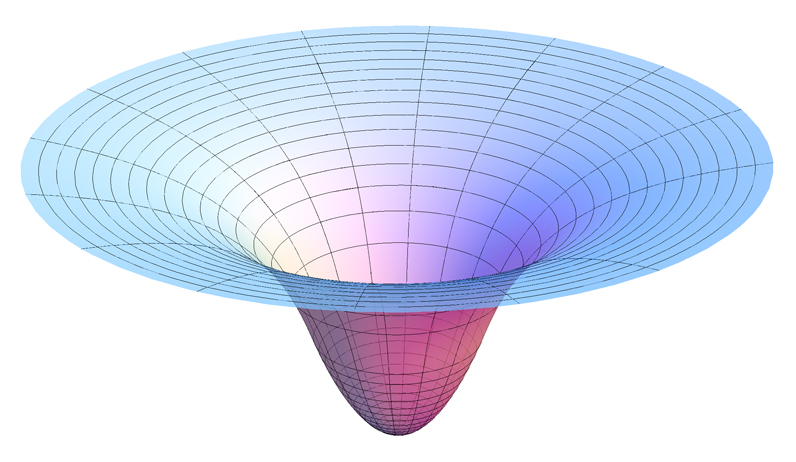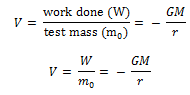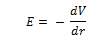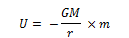The gravitational potential at a point in a gravitational field is defined as the amount of work done in bringing a body of unit mass from infinity to that point without acceleration.

Gravitational potential at a point,Gravitational intensity (I) is related to gravitational potential (V) at a point by the relation,Gravitational potential energy of a body

### Gravitational potential energy of a body

at a point in the gravitational field of another body is defined as the amount of work done in bringing the given body from infinity to that point without acceleration.

Gravitational potential energy U = gravitational potential × mass of bodywhere U is taken to be zero at r → ∞. The total potential energy of a system of particles is the sum of energies for all pairs of particles, with each pair represented by a term of the form given by above equation. This prescription follows from the principle of superposition.

If an isolated system consists of a particle of mass m moving at a speed v in the vicinity of a massive body of mass M, the total mechanical energy of the particle is given by

E = K.E + P.E

That is, the total mechanical energy is the sum of the kinetic and potential energies. The total energy is a constant of motion.

Click on a star to rate it!

Average rating 0 / 5. Vote count: 0

No votes so far! Be the first to rate this post.

As you found this post useful...